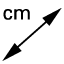G.GCI.4 Constructing Tangents

DIRECTIONS:

In the applet below, note the circle with center A. You can alter the radius by dragging the orange point on the circle. Point B is a point on the circle. Point C is a point that lies outside the circle. Feel free to alter the locations of any of these 4 points at any time. 1) Use the TANGENTStool to construct a line through B that is tangent to the circle. 2) Use the TANGENTS tool to construct a line passing through C that is tangent to the circle. What do you notice? 3) Use the INTERSECTtool to plot the point(s) at which these tangents intersect the circle. GeoGebra should name these points D and E. Further directions appear below the applet.
4) Construct 2 radii with endpoint A (obviously) and other endpoint located at each of the points you constructed in step (3).

5)

Measure the angle at which each radius meets each tangent. What do you notice?

6)

Use the DISTANCE OR LENGTHtool to measure the distance from C to D and the distance from C to E. What do you notice?

When you're done (or if you're unsure of something) feel free to check by watching the brief silent screencast below the applet.